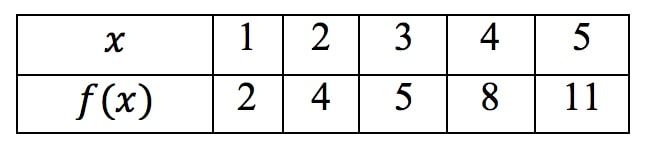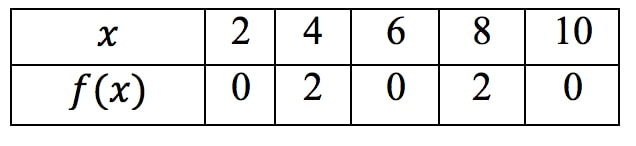# Estimating Derivatives from a table

### Estimating Derivatives from a table

#### Lessons

Recall when finding the slope of two points, we use the formula

$m = \frac{y_{2}-y_{1}}{x_{2}-x_{1}}$

Since the slope is the derivative, we can actually use this formula to estimate derivatives from a table.

We will just readjust the slope formula to look like this:

$f'(a) \approx \frac{f(a+h) - f(a)}{h}$

Use this when estimating the slope of the very first point of the table

$f'(a) \approx \frac{f(a+h) - f(a-h)}{2h}$

Use this when estimating the slope of middle points of the table

$f'(a) \approx \frac{f(a) - f(a-h)}{h}$

Use this when estimating the slope of the very last point of the table

Note that we will not use these formulas in the videos. We will just use the simple slope formula.

• Introduction
Estimating Derivatives from a table Overview:

• 1.
Estimating Derivatives

Use the table below to estimate the following derivatives as accurately as possible:a)
$f'(1)$

b)
$f'(3)$

c)
$f'(5)$

• 2.
Use the table below to estimate the following derivatives as accurately as possible:a)
$f'(2)$

b)
$f'(6)$

c)
$f'(10)$